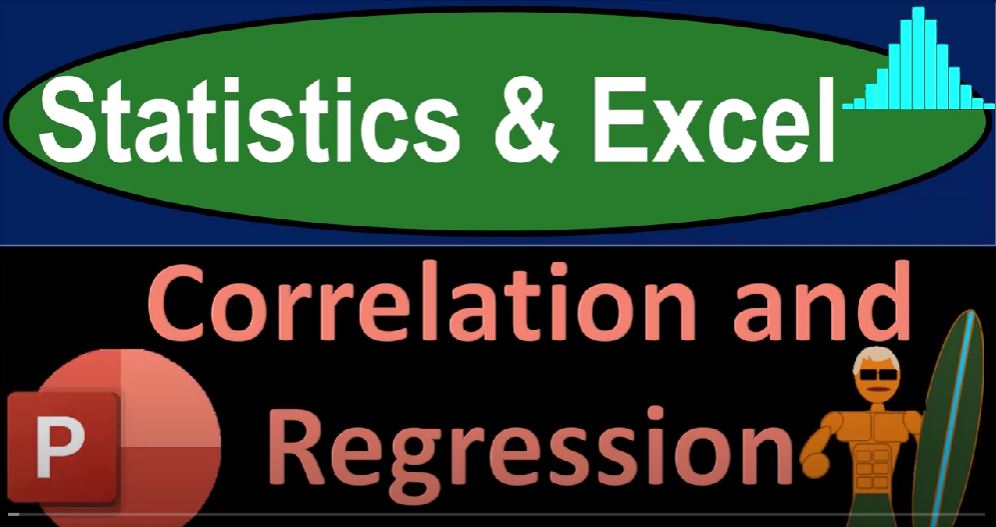## Correlation and Regression 1710 Statistics & Excel

In the realm of statistics and data analysis, the concepts of correlation and regression play a pivotal role in unveiling relationships between variables. In this blog post, we will dive into the world of statistics and Excel, exploring the nuances of correlation, the importance of avoiding the correlation-causation fallacy, and how regression can be a powerful tool for making predictions.

## Understanding Correlation:

Correlation is the measurement of the strength and direction of the linear relationship between two variables. A common phrase associated with correlation is “correlation does not necessarily mean causation,” emphasizing the need to critically analyze the data. While this phrase is widely known, its significance often gets overlooked.

### Recap of Prior Sections:

Before delving into correlation, it’s essential to recap the descriptive methods used to analyze datasets, including mean, median, mode, quartiles, and visual representations like histograms. These tools help us understand the distribution and spread of data, preparing us for the exploration of correlations.

### Types of Correlations:

1. Positive Correlation: Both variables increase together. Illustrated with the example of the number of hens and the corresponding eggs produced.
2. Negative Correlation: One variable increases while the other decreases. Examined through the relationship between age and batting averages.
3. Correlation Coefficient (R): A mathematical representation ranging from -1 to 1, indicating the strength and direction of the correlation. The formula involves z-scores and standard deviations.

### Extreme Correlation Examples:

• Perfect Positive Correlation: Illustrated with the conversion of feet to inches.
• Perfect Negative Correlation: Demonstrated with the distance traveled and the distance remaining during a trip.
• Zero Correlation: Shown with a flat trendline, indicating no linear correlation.

### Scatter Plots and Identifying Patterns:

Scatter plots visually represent the relationship between two variables, aiding in the identification of linear patterns. Understanding these patterns helps discern correlations and potential cause-and-effect relationships.

## The Role of Regression:

Regression comes into play when we seek to make predictions based on the identified relationship between variables. The focus is often on simple linear regression, where one independent variable predicts the value of a dependent variable.

### Residuals and Least Squares Method:

Residuals represent the differences between actual and predicted values. The goal of regression is to minimize these residuals, achieved through the least squares method. This method optimally fits a line through the data points.

### Multiple Regression:

As systems become more complex, multiple regression allows for the consideration of multiple independent variables to create a more accurate model. This is particularly useful in predicting outcomes like house prices, where factors such as size, location, and age come into play.

## Correlation vs. Causation:

The mantra “correlation does not equal causation” is a crucial reminder. Misinterpretation of correlations can lead to incorrect calculations and misguided actions. Specious correlations, or relationships that seem meaningful but are coincidental or influenced by external factors, can misguide analyses.

### Examples of Specious Correlations:

1. Ice Cream Sales and Shark Attacks: Highlighting the importance of critically evaluating correlations.
2. Pool Drownings and Nicolas Cage Films: Emphasizing the absurdity of drawing causal relationships from random correlations.

## Conclusion:

In the realm of statistics and Excel, understanding correlation and regression is essential for making informed decisions. By recognizing the limitations of correlation, avoiding the correlation-causation fallacy, and leveraging regression for predictions, we can navigate the complex landscape of data analysis with confidence. As we embark on practical examples and exercises in the following sections, the power of statistical tools and Excel will become even more evident.